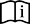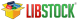Time Library

The Time Library contains functions and type definitions for time calculations in the UNIX time format which counts the number of seconds since the "epoch". This is very convenient for programs that work with time intervals: the difference between two UNIX time values is a real-time difference measured in seconds.

What is the epoch?
Originally it was defined as the beginning of 1970 GMT. (January 1, 1970 Julian day) GMT, Greenwich Mean Time, is a traditional term for the time zone in England.

The TimeStruct type is a structure type suitable for time and date storage. Type declaration is contained in __Time.h which can be found in the mikroC PRO for PIC Time Library Demo example folder.

Time_dateToEpoch

Prototype long Time_dateToEpoch(TimeStruct *ts); Number of seconds since January 1, 1970 0h00mn00s. This function returns the UNIX time : number of seconds since January 1, 1970 0h00mn00s. Parameters : ts: time and date value for calculating UNIX time. Nothing. #include "timelib.h" ... TimeStruct ts1; long epoch; ... /* * what is the epoch of the date in ts ? */ epoch = Time_dateToEpoch(&ts1);

Time_epochToDate

Prototype void Time_epochToDate(long e, TimeStruct *ts); Nothing. Converts the UNIX time to time and date. Parameters : e: UNIX time (seconds since UNIX epoch) ts: time and date structure for storing conversion output Nothing. #include "timelib.h" ... TimeStruct ts2; long epoch; ... /* * what date is epoch 1234567890 ? */ epoch = 1234567890; Time_epochToDate(epoch, &ts2);

Time_dateDiff

Prototype long Time_dateDiff(TimeStruct *t1, TimeStruct *t2); Time difference in seconds as a signed long. This function compares two dates and returns time difference in seconds as a signed long. Result is positive if t1 is before t2, result is null if t1 is the same as t2 and result is negative if t1 is after t2. Parameters : t1: time and date structure (the first comparison parameter) t2: time and date structure (the second comparison parameter)Note : This function is implemented as macro in the timelib.h header file which can be found in the mikroC PRO for PIC Time Library Demo example folder. Nothing. #include "timelib.h" ... TimeStruct ts1, ts2; long diff; ... /* * how many seconds between these two dates contained in ts1 and ts2 buffers? */ diff = Time_dateDiff(&ts1, &ts2);

Library Example

Demonstration of Time library routines usage for time calculations in UNIX time format.

#include        "timelib.h"

TimeStruct ts1, ts2 ;
long epoch ;
long diff ;

void main() {

ts1.ss = 0 ;
ts1.mn = 7 ;
ts1.hh = 17 ;
ts1.md = 23 ;
ts1.mo = 5 ;
ts1.yy = 2006 ;

/*
* What is the epoch of the date in ts ?
*/
epoch = Time_dateToEpoch(&ts1) ;       //  1148404020

/*
* What date is epoch 1234567890 ?
*/
epoch = 1234567890 ;
Time_epochToDate(epoch, &ts2) ;       //  {0x1E, 0x1F,0x17, 0x0D, 0x04, 0x02, 0x07D9}

/*
* How much seconds between this two dates ?
*/
diff = Time_dateDiff(&ts1, &ts2) ;    //  86163870
}
Find them on